f
Neutrium

# Flow Rate

Pressure Loss in Hoses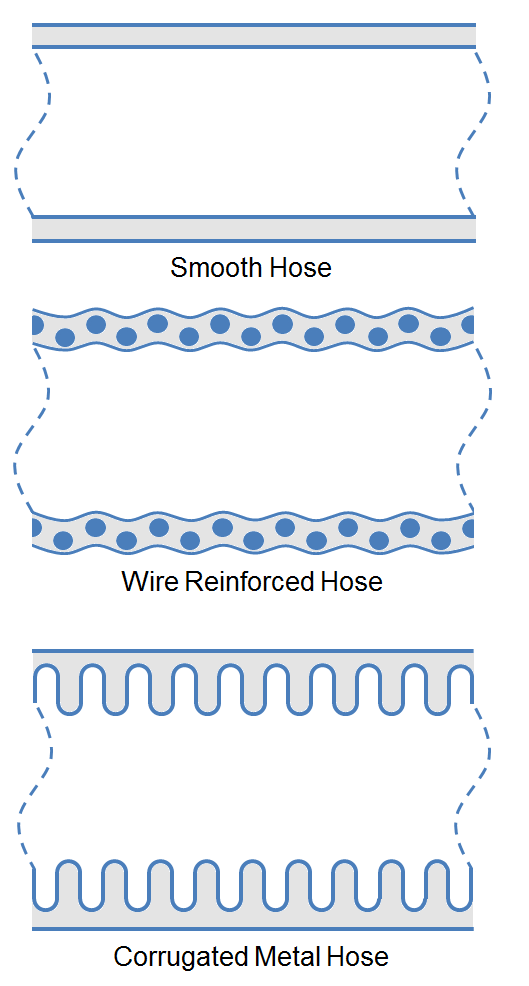The Pressure loss through a hose is often approximated using coarse heuristics, but utilization of more accurate correlations increase the efficiency of pump and piping designs. This article presents more accurate methods to estimate the pressure loss in various type of hoses using multiples of the pipe length. Methods of estimating pressure loss caused by couplings, curves and coiled hose are also detailed.

Calculating Thermal Relief Flow Rates

For long sections of pipe, the thermal expansion of trapped liquid can be significant. It is often required that the increase in volume of the fluid be determined in order to select suitable thermal relief valves to protect the integrity of the pipework. This article details how to calculate the required relief flow rate to prevent over pressure due to thermal expansion.

Pump Specific Speed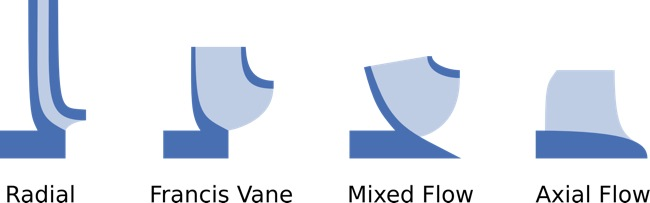Pump Specific Speed, NS is a method of characterising a pump duty by head, flow rate and rotational speed. Pump specific speed may be used to determine an appropriate pump design for a given application when choosing between axial, radial or multistage centrifugal designs or positive displacement pumps.

Pressure Loss from Fittings - Expansion and Reduction in Pipe Size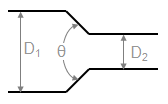This article provides methods to calculate the K-value (Resistance Coefficient) for determining the pressure loss cause by changes in the area of a fluid flow path. These types of pressure drops are highly dependent on the geometry and are not usually covered in simple pressure loss estimation schemes (such as a single k-value, equivalent length etc.)

Pressure Loss from Fittings - 3K Method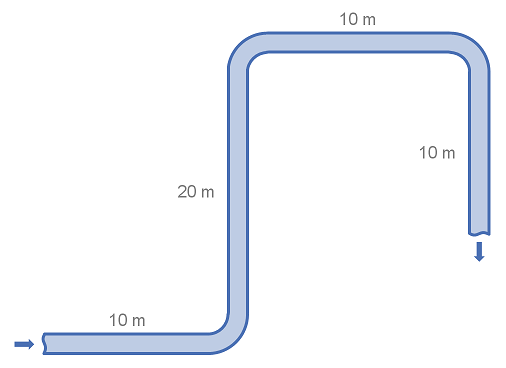Fittings such as elbows, tees, valves and reducers represent a significant component of the pressure loss in most pipe systems. This article details the calculation of pressure losses through pipe fittings and some minor equipment using the 3K method.

Pressure Loss from Fittings - 2K Method

Fittings such as elbows, tees, valves and reducers represent a significant component of the pressure loss in most pipe systems. This article details the calculation of pressure losses through pipe fittings and some minor equipment using the 2K method.

Pressure Loss from Fittings - Excess Head (K) Method

Fittings such as elbows, tees, valves and reducers represent a significant component of the pressure loss in most pipe systems. This article details the calculation of pressure losses through pipe fittings and some minor equipment using the K-value method, also known as the Resistance Coefficient, Velocity Head, Excess Head or Crane method.

Pressure Loss from Fittings - Equivalent Length MethodFittings such as elbows, tees and valves represent a significant component of the pressure loss in most pipe systems. This article details the calculation of pressure losses through pipe fittings and some minor equipment using the equivalent length method. The strength of the equivalent length method is that it is very simple to calculate. The weakness of the equivalent length method is that it is not as accurate as other methods unless very detailed tabulated data is available.

Converting Between Cv, Kv and K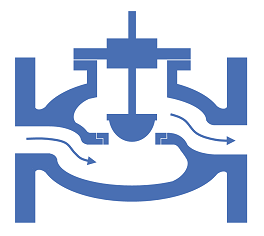There are several common ways to express the losses caused by pipe fittings and equipment. Depending on the calculation programs or methods available and engineer may require to convert between one form or another. This article details the equations required to convert between the resistance coefficient and flow coefficient methods (K, Cv and Kv).

Pressure Loss from Fittings in Pipe Summary

Fittings such as elbows, tees, valves and reducers represent a significant component of the pressure loss in most pipe systems. This article discusses the differences between several popular methods for determining the pressure loss through fittings. The methods discussed for fittings are: the equivalent length method, the K method (velocity head method or resistance coefficient method), the two-K method and the three-K method. In this article we also discuss method for calculating pressure loss through pipe size changes as well as control valves.